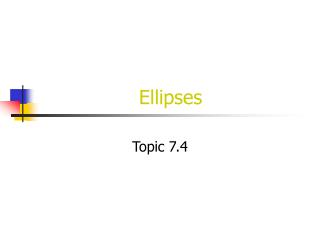DownloadDownload PresentationEllipses

# Ellipses

Download Presentation## Ellipses

- - - - - - - - - - - - - - - - - - - - - - - - - - - E N D - - - - - - - - - - - - - - - - - - - - - - - - - - -
##### Presentation Transcript

1. Ellipses Topic 7.4

2. Definitions • Ellipse: set of all points where the sum of the distances from the foci is constant • Major Axis: axis on which the foci lie; the longer axis of symmetry • Minor Axis: the shorter axis of symmetry

3. Two Standard Equations • Horizontal Ellipse: • Foci: • Vertical Ellipse: • Foci:

4. Writing in Standard Form • Complete the square for both the x-terms and y-terms and move the constant to the other side of the equation • Divide all terms by the constant

5. Example: Group terms Complete the square Simplify each group Divide by constant

6. Graphing the ellipse • Put equation in standard form • Graph the center (h, k) • Graph the foci (look at the equation to determine your direction) • Graph a units and –a units from the center to get the end points (horizontally if under x, vertically if under y) • Connect the end points!

7. Example: 1) Graph Center 2) Graph Foci 3) Graph Endpoints 4) Graph Ellipse